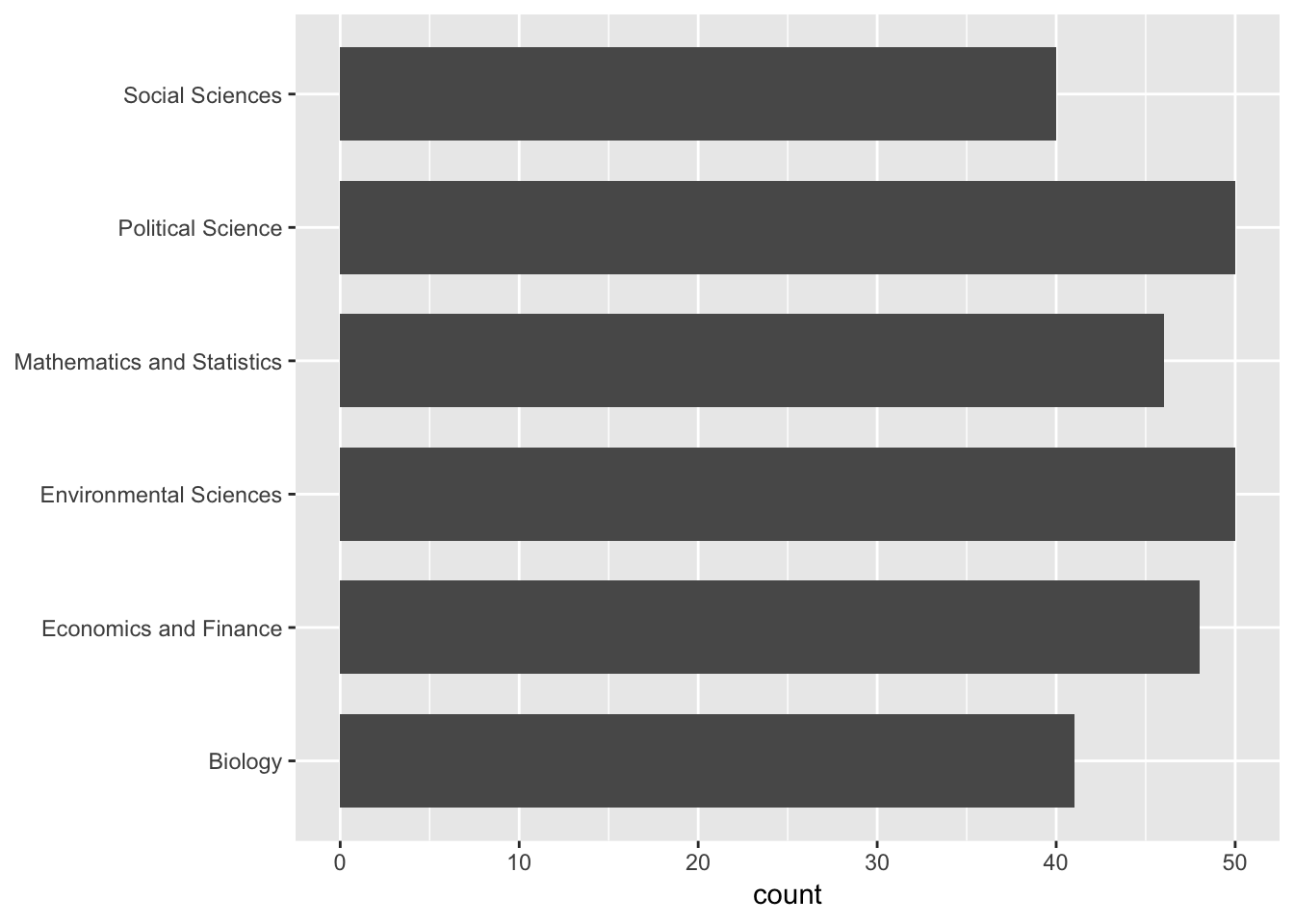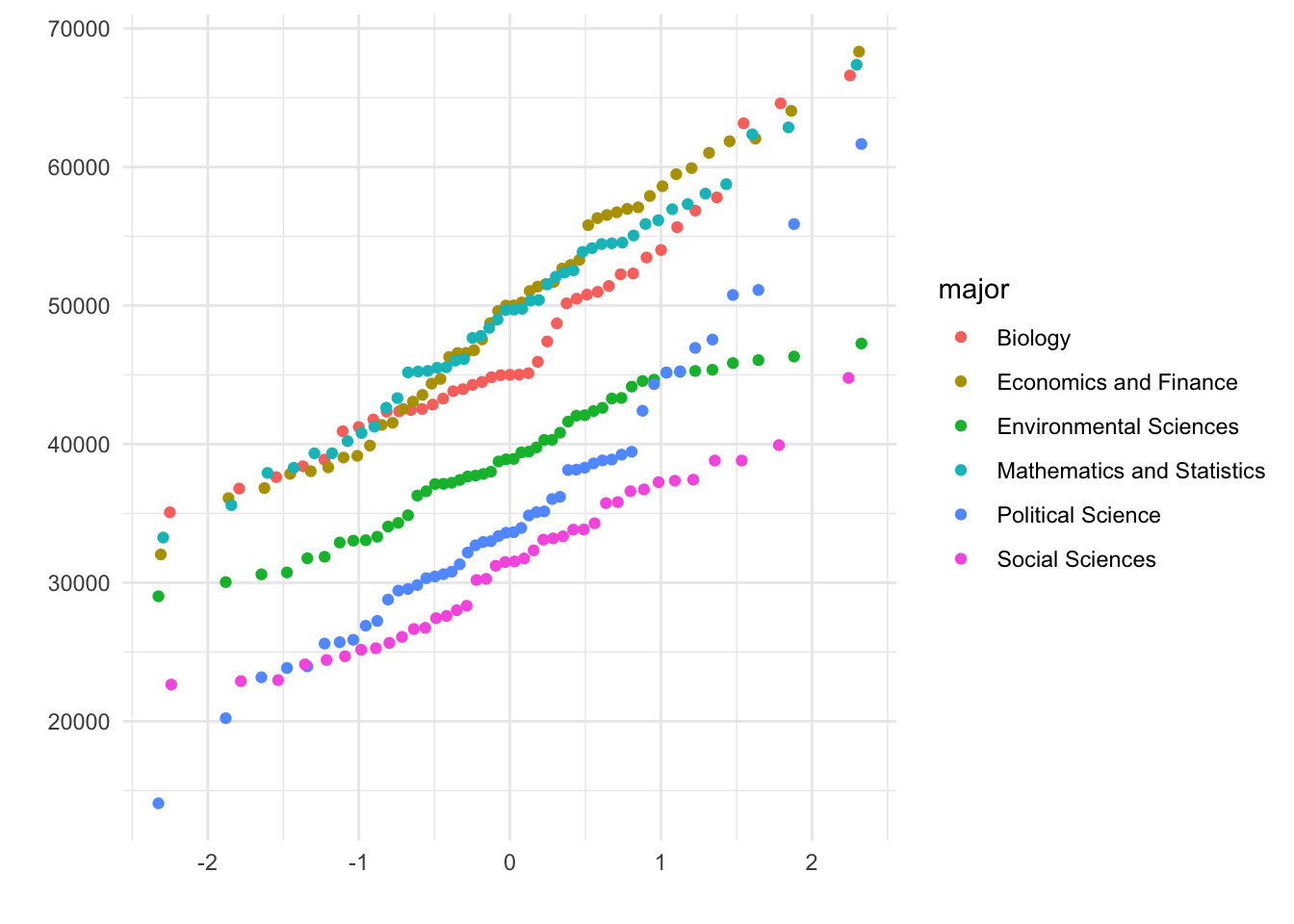The purpose of an one-way ANOVA hypothesis test is to compare $$k$$ population/group means, $$\mu_1, \mu_1, ...,\mu_k$$. The following assumptions have to be fulfilled in order to apply a one-way ANOVA (Weiss 2010):

• Random samples
• Independent samples
• For each population, the variable under consideration is normally distributed.
• The standard deviations of the variable under consideration are the same for all the populations.

A one-way ANOVA hypothesis test follows the same step-wise procedure as other hypothesis tests. $\begin{array}{l} \hline \ \text{Step 1} & \text{State the null hypothesis } H_0 \text{ and alternative hypothesis } H_A \text{.}\\ \ \text{Step 2} & \text{Decide on the significance level, } \alpha\text{.} \\ \ \text{Step 3} & \text{Compute the value of the test statistic.} \\ \ \text{Step 4} &\text{Determine the p-value.} \\ \ \text{Step 5} & \text{If } p \le \alpha \text{, reject }H_0 \text{; otherwise, do not reject } H_0 \text{.} \\ \ \text{Step 6} &\text{Interpret the result of the hypothesis test.} \\ \hline \end{array}$

#### One-way ANOVA Hypothesis Test: An example

In order to get some hands-on experience we apply the one-way ANOVA hypothesis test in an exercise. Therefore we load the students data set. You may download the students.csv file here. Import the data set and assign a proper name to it.

students <- read.csv("https://userpage.fu-berlin.de/soga/200/2010_data_sets/students.csv")

The students data set consists of 8239 rows, each of them representing a particular student, and 16 columns, each of them corresponding to a variable/feature related to that particular student. These self-explaining variables are: stud.id, name, gender, age, height, weight, religion, nc.score, semester, major, minor, score1, score2, online.tutorial, graduated, salary.

In order to showcase the one-way ANOVA hypothesis test we examine the mean annual salary of graduates, grouped by their major study subject. To make it clear, in this example the classification/grouping is done by one variable, the major variable, the so-called factor, thus we are conducting a one-way ANOVA. In this exercise we want to test if the mean annual salary of graduates is different among graduates of different study subjects.

#### Data exploration and preparation

We start our data analysis with the random sampling process. We randomly sample 275 students from the data set using the sample() function in R. We want to make sure to sample only from graduated students, that is why we subset the data beforehand with the subset() function in R. Further, we reduce our data set to the two variables of interest, the categorical variable major and the numeric variable salary. Then we display the first 10 rows of the data set.

graduated <- subset(students, graduated == 1)

n <- 275
data.idx <- sample(x = 1:nrow(graduated), size = n)
head(data, 10)
##                           major   salary
## 1619     Environmental Sciences 46320.61
## 8183 Mathematics and Statistics 46139.35
## 4076 Mathematics and Statistics 58760.82
## 7378      Economics and Finance 46578.71
## 7291                    Biology 44487.23
## 4022          Political Science 29832.22
## 8229 Mathematics and Statistics 33259.70
## 1623 Mathematics and Statistics 48991.98
## 6250 Mathematics and Statistics 49766.16
## 1384            Social Sciences 26744.06

Just for a matter of interest we visualize the counts for each of the 6 different study subjects in our sample by plotting a bar plot. We us the ggplot2 library for plotting.

library(ggplot2)
p <- ggplot(data, aes(major)) +
geom_bar(width=.7) +
coord_flip() +
theme(axis.title.y=element_blank())
pWe see that the different study subjects are not equally distributed in our sample, but that is okay.

Before we start with the hypothesis test, we check if the assumptions for the one-way ANOVA hypothesis test are fulfilled. The samples are random and independent samples. That is fine. We check the normality assumption by plotting a normal probability plot (Q-Q plots) for each grouped variable.

p <- qplot(sample = salary,
data = data,
color=major) +
theme_minimal()
pWell, not perfect but we may assume that the data for each group falls roughly on a straight line.

Next, we test the assumption of equal standard deviations. Therefore we calculate the standard deviation for each group. The programming language R provides some excellent functions to calculate statistical parameters across different groupings of a data set. These functions refer to the apply() function family. For our example we use the tapply() function to calculate the standard deviations for each group.

#calculate standart deviation
sd.groups <- tapply(X = data$salary, INDEX = data$major, FUN = sd)
sd.groups
##                    Biology      Economics and Finance
##                   7418.253                   8521.318
##     Environmental Sciences Mathematics and Statistics
##                   4983.206                   7532.158
##          Political Science            Social Sciences
##                   9140.026                   5532.529

As a rule of thumb, we consider the equal standard deviations assumption as fulfilled, if the ratio of the largest to the smallest sample standard deviation is less than 2 (Weiss 2010).

#calculate ratio of the largest to the smallest sample standard deviation
ratio <- max(as.vector(sd.groups)) / min(as.vector(sd.groups))
ratio
##  1.834166

The ratio of the largest to the smallest sample standard deviation is 1.834. That is close to the threshold of 2, but for our toy example still acceptable. Thus, we conclude that the assumptions are fulfilled.

Note that one-way ANOVA is robust to moderate violations of normality assumption and the equal standard deviations assumption (Weiss 2010).

#### Hypothesis Testing

In order to conduct the one-way ANOVA hypothesis test we follow the step-wise implementation procedure for hypothesis testing.

Step 1: State the null hypothesis $$H_0$$ and alternative hypothesis $$H_A$$

The null hypothesis states that the mean annual salary is equal among all groups of graduates.

$H_0: \quad \mu_1=\mu_2=\mu_3=\mu_4=\mu_5=\mu_6$

Alternative hypothesis $H_A: \quad \text{Not all the means are equal}$

Step 2: Decide on the significance level, $$\alpha$$

$\alpha = 0.01$

alpha <- 0.01

Step 3 and 4: Compute the value of the test statistic and the p-value.

In order to calculate the $$F$$ test statistic, we need to determine several quantities beforehand. For illustration purposes we manually compute the $$F$$ test statistic in R. Step by step we populate the ANOVA table until we finally get the $$F$$ test statistic and consequently the p-value.

$\begin{array}{|l|c|} \hline \ \text{Source} & df & \text{Sum of Squares }(SS) & \text{Mean Squares }(MS) & F\text{-statistic} & p\text{-value}\\ \hline \ \text{Group} & k-1 & SSG & MSG=\frac{SSG}{k-1} & F = \frac{MSG}{MSE} & p\\ \ \text{Error} & n-k & SSE & MSE=\frac{SSE}{n-k} & & \\ \hline \ \text{Total} & n-1 & SST & & & \\ \hline \end{array}$ We start with the first column and calculate the degrees of freedom.

k <- length(levels(data$major)) n <- nrow(data) df.G <- k - 1 df.G ##  5 df.E <- n - k df.E ##  269 df.T <- n - 1 df.T ##  274 $\begin{array}{|l|c|} \hline \ \text{Source} & df & \text{Sum of Squares }(SS) & \text{Mean Squares }(MS) & F\text{-statistic} & p\text{-value}\\ \hline \ \text{Group} & 5 & SSG & MSG=\frac{SSG}{k-1} & F = \frac{MSG}{MSE} & p\\ \ \text{Error} & 269 & SSE & MSE=\frac{SSE}{n-k} & & \\ \hline \ \text{Total} & 274 & SST & & & \\ \hline \end{array}$ Further, we calculate the three sums of squares, $$SST$$, $$SSG$$, and $$SSE$$. Recall the equations from above. $SST = \sum_{i=1}^n(x_i-\bar x)^2\text{,}$ where $$x_i$$ corresponds to the observations in the samples and $$\bar x$$ to the overall mean of all samples. # calculate overall mean x.bar <- mean(data$salary)

# observation data
xi <- data$salary # calculate SST SST <- sum((xi - x.bar)^2) SST ##  28530785099 $SSG = \sum_{i=1}^n n_j(\bar x_i-\bar x)^2$ Here, $$n_j$$ denotes the sample size for group $$j$$, $$\bar x_i$$ denotes the mean of group $$j$$ and $$\bar x$$ denotes to the overall mean of all samples. # calculate sample size for each group n.j <- tapply(X = data$salary, INDEX = data$major, FUN = length) # calculate mean for each group xi.bar <- tapply(X = data$salary, INDEX = data$major, FUN = mean) # calculate SSG SSG <- sum(n.j * (xi.bar - x.bar)^2) SSG ##  13859762704 $SSE = \sum_{i=1}^n (n_j-1)s_j^2\text{,}$ where $$n_j$$ denotes the sample size for group $$j$$ and $$s^2_j$$ the variance of group $$j$$. # calculate standart deviation for each group s2.j <- tapply(X = data$salary, INDEX = data\$major, FUN = sd)

# calculate SSE
SSE <- sum((n.j-1)*s2.j^2)
SSE
##  14671022395
# alternatively one may calculate SSE this way
# SSE = SST - SSG

$\begin{array}{|l|c|} \hline \ \text{Source} & df & \text{Sum of Squares }(SS) & \text{Mean Squares }(MS) & F\text{-statistic} & p\text{-value}\\ \hline \ \text{Group} & 5 & 1.3859763\times 10^{10} & MSG=\frac{SSG}{k-1} & F = \frac{MSG}{MSE} & p\\ \ \text{Error} & 269 & 1.4671022\times 10^{10} & MSE=\frac{SSE}{n-k} & & \\ \hline \ \text{Total} & 274 & 2.8530785\times 10^{10} & & & \\ \hline \end{array}$ Now we calculate the two measures of mean variability, $$MSG$$, and $$MSE$$.

# calculate MSG
MSG <- SSG/(k-1)
MSG
##  2771952541
# calculate MSE
MSE <- SSE/(n-k)
MSE
##  54539117

$\begin{array}{|l|c|} \hline \ \text{Source} & df & \text{Sum of Squares }(SS) & \text{Mean Squares }(MS) & F\text{-statistic} & p\text{-value}\\ \hline \ \text{Group} & 5 & 1.3859763\times 10^{10} & 2.7719525\times 10^{9} & F = \frac{MSG}{MSE} & p\\ \ \text{Error} & 269 & 1.4671022\times 10^{10} & 5.4539117\times 10^{7} & & \\ \hline \ \text{Total} & 274 & 2.8530785\times 10^{10} & & & \\ \hline \end{array}$

Finally we obtain the $$F$$-statistics by the ratio of $$MSG$$ and $$MSE$$.

Fstat <- MSG/MSE
Fstat
##  50.82504

$\begin{array}{|l|c|} \hline \ \text{Source} & df & \text{Sum of Squares }(SS) & \text{Mean Squares }(MS) & F\text{-statistic} & p\text{-value}\\ \hline \ \text{Group} & 5 & 1.3859763\times 10^{10} & 2.7719525\times 10^{9} & 50.8250355 & p\\ \ \text{Error} & 269 & 1.4671022\times 10^{10} & 5.4539117\times 10^{7} & & \\ \hline \ \text{Total} & 274 & 2.8530785\times 10^{10} & & & \\ \hline \end{array}$

In the last step we calculate the p-value by calling the pf() function in R. Recall how to calculate the degrees of freedom.

$df = (k-1, n-k)$

Because the null hypothesis is rejected only when the test statistic, $$F$$, is too large, a one-way ANOVA test is always right tailed.

df1 = k-1
df2 = n-k

p.value <- pf(q = Fstat, df1 = df1, df2 = df2, lower.tail = FALSE)
p.value
##  5.746974e-37

$\begin{array}{|l|c|} \hline \ \text{Source} & df & \text{Sum of Squares }(SS) & \text{Mean Squares }(MS) & F\text{-statistic} & p\text{-value}\\ \hline \ \text{Group} & 5 & 1.3859763\times 10^{10} & 2.7719525\times 10^{9} & F = 50.8250355 & 5.7469735\times 10^{-37}\\ \ \text{Error} & 269 & 1.4671022\times 10^{10} & 5.4539117\times 10^{7} & & \\ \hline \ \text{Total} & 274 & 2.8530785\times 10^{10} & & & \\ \hline \end{array}$

Step 5: If $$p \le \alpha$$, reject $$H_0$$; otherwise, do not reject $$H_0$$.

p.value <= alpha
##  TRUE

The p-value is less than the specified significance level of 0.01; we reject $$H_0$$. The test results are statistically significant at the 1% level and provide very strong evidence against the null hypothesis.

Step 6: Interpret the result of the hypothesis test.

$$p = 5.7469735\times 10^{-37}$$. At the 1% significance level, the data provides very strong evidence to conclude that at least one pair of group means is different from each other.

#### Hypothesis Testing in R

We just completed a one-way ANOVA hypothesis test in R manually. Awesome, but now we redo that example and make use of the R machinery to obtain the same result as above by just one line of code!

In order to conduct a one-way ANOVA hypothesis test in R we apply the aov() function. The aov() function expects the so-called formula-notation, thus we provide our data by separating our two variables of interest by ~. Further, we provide a data frame in which the variables specified in the formula are found.

anova <- aov(salary ~ major, data = data)
summary(anova)
##              Df    Sum Sq   Mean Sq F value Pr(>F)
## major         5 1.386e+10 2.772e+09   50.83 <2e-16 ***
## Residuals   269 1.467e+10 5.454e+07
## ---
## Signif. codes:  0 '***' 0.001 '**' 0.01 '*' 0.05 '.' 0.1 ' ' 1

Worked out fine! Compare the output of the aov() function with our result from above. Again, we may conclude that at the 1% significance level, the data provides very strong evidence to conclude that at least one pair of group means is different from each other.